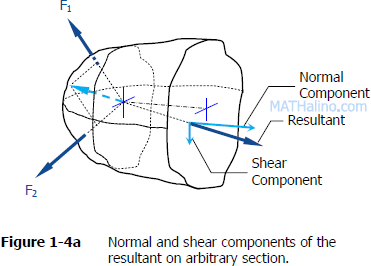04-05 Stiffness and strength of timber beam Jhun Vert Fri, 05/08/2020 - 03:09 pm

Problem 4
The stiffness of a rectangular beam is proportional to the breadth and the cube of the depth. Find the shape of the stiffest beam that can be cut from a log of a given size.

Problem 5
The strength of rectangular beam is proportional to the breadth and the square of the depth. Find the shape of the strongest beam that can be cut from a log of given size.

18 - 20 Rectangular beam in maxima and minima problems Jhun Vert Mon, 05/04/2020 - 10:23 pm

Problem 18
The strength of a rectangular beam is proportional to the breadth and the square of the depth. Find the shape of the largest beam that can be cut from a log of given size.

Problem 19
The stiffness of a rectangular beam is proportional to the breadth and the cube of the depth. Find the shape of the stiffest beam that can be cut from a log of given size.

Problem 20
Compare for strength and stiffness both edgewise and sidewise thrust, two beams of equal length, 2 inches by 8 inches and the other 4 inches by 6 inches (See Problem 18 and Problem 19 above). Which shape is more often used for floor joist? Why?

Solution to Problem 581 | Design for Flexure and Shear Jhun Vert Wed, 04/22/2020 - 11:44 pm

Problem 581
A laminated beam is composed of five planks, each 6 in. by 2 in., glued together to form a section 6 in. wide by 10 in. high. The allowable shear stress in the glue is 90 psi, the allowable shear stress in the wood is 120 psi, and the allowable flexural stress in the wood is 1200 psi. Determine the maximum uniformly distributed load that can be carried by the beam on a 6-ft simple span.

## Solution to Problem 572 | Horizontal Shearing Stress

Problem 572
The T section shown in Fig. P-572 is the cross-section of a beam formed by joining two rectangular pieces of wood together. The beam is subjected to a maximum shearing force of 60 kN. Show that the NA is 34 mm from the top and the INA = 10.57 × 106 mm4. Using these values, determine the shearing stress (a) at the neutral axis and (b) at the junction between the two pieces of wood.Solution to Problem 527 | Flexure Formula Jhun Vert Wed, 04/22/2020 - 10:59 am

Problem 527
In Prob. 526, if the load on the overhang is 600 lb/ft and the overhang is x ft long, find the maximum values of P and x that can be used simultaneously.Solution to Problem 526 | Flexure Formula Jhun Vert Wed, 04/22/2020 - 10:51 am

Problem 526
A wood beam 6 in wide by 12 in deep is loaded as shown in Fig. P-526. If the maximum flexural stress is 1200 psi, find the maximum values of wo and P which can be applied simultaneously?## Solution to Problem 129 Bearing Stress

Problem 129A 7/8-in.-diameter bolt, having a diameter at the root of the threads of 0.731 in., is used to fasten two timbers together as shown in Fig. P-129. The nut is tightened to cause a tensile stress of 18 ksi in the bolt. Compute the shearing stress in the head of the bolt and in the threads. Also, determine the outside diameter of the washers if their inside diameter is 9/8 in. and the bearing stress is limited to 800 psi.

Given:
Diameter of bolt = 7/8 inch
Diameter at the root of the thread (bolt) = 0.731 inch
Inside diameter of washer = 9/8 inch
Tensile stress in the nut = 18 ksi
Bearing stress = 800 psi

Solution to Problem 123 Shear Stress Jhun Vert Mon, 04/20/2020 - 01:37 pm

Problem 123
A rectangular piece of wood, 50 mm by 100 mm in cross section, is used as a compression block shown in Fig. P-123. Determine the axial force P that can be safely applied to the block if the compressive stress in wood is limited to 20 MN/m2 and the shearing stress parallel to the grain is limited to 5MN/m2. The grain makes an angle of 20° with the horizontal, as shown. (Hint: Use the results in Problem 122.)Solution to Problem 122 Shear Stress Jhun Vert Mon, 04/20/2020 - 01:33 pm

Problem 122
Two blocks of wood, width w and thickness t, are glued together along the joint inclined at the angle θ as shown in Fig. P-122. Using the free-body diagram concept in Fig. 1-4a, show that the shearing stress on the glued joint is τ = P sin 2θ / 2A, where A is the cross-sectional area.Solution to Problem 110 Normal Stress Jhun Vert Mon, 04/20/2020 - 12:38 pm

Problem 110
A 12-inches square steel bearing plate lies between an 8-inches diameter wooden post and a concrete footing as shown in Fig. P-110. Determine the maximum value of the load P if the stress in wood is limited to 1800 psi and that in concrete to 650 psi.